# dynamics chapt 1 .pptx

20 de Dec de 2022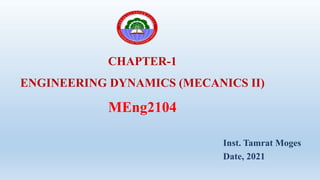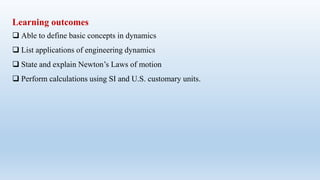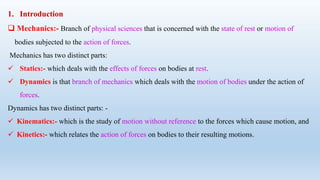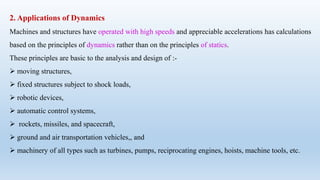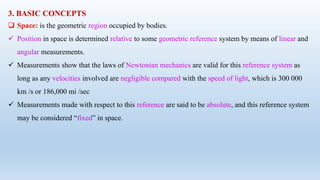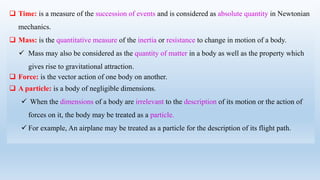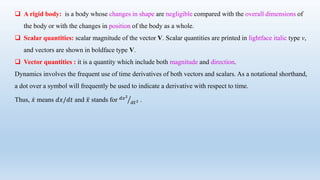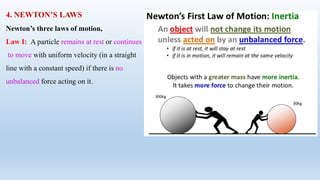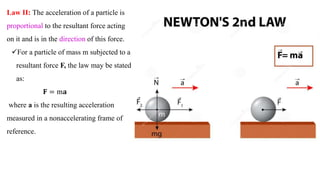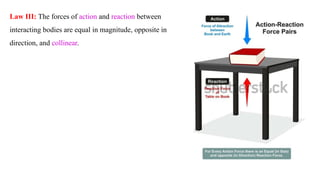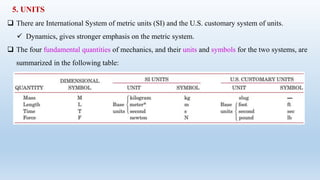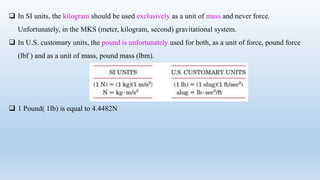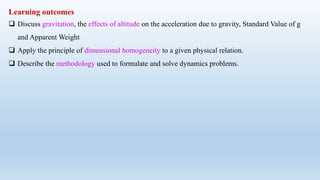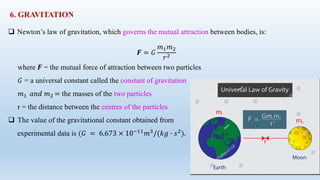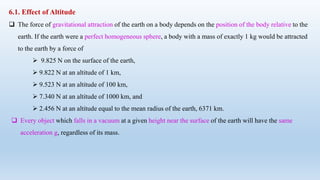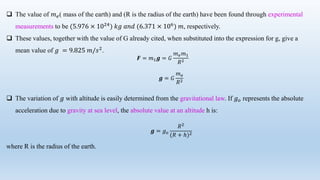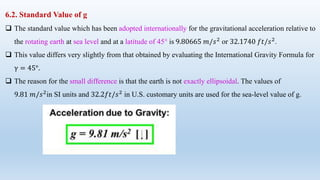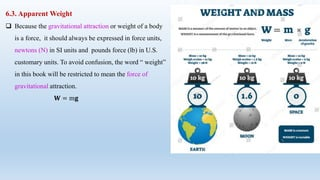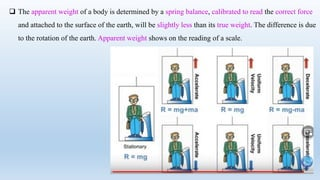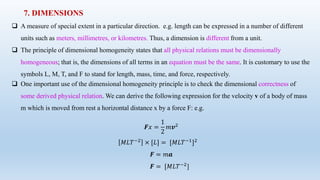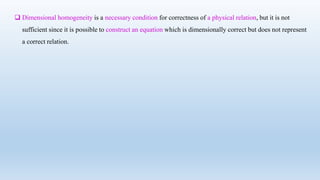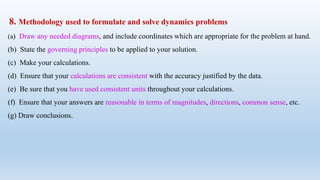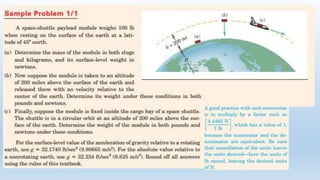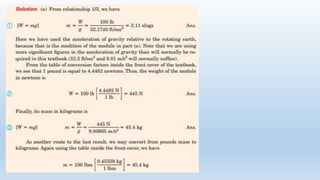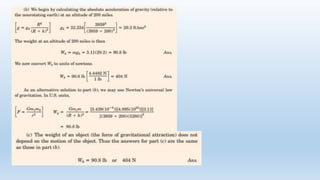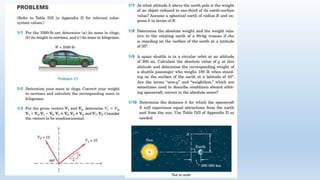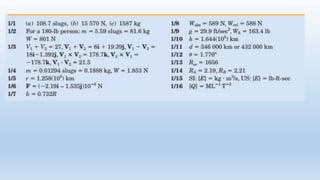1 de 30

### dynamics chapt 1 .pptx

• 1. CHAPTER-1 ENGINEERING DYNAMICS (MECANICS II) MEng2104 Inst. Tamrat Moges Date, 2021
• 2. Learning outcomes  Able to define basic concepts in dynamics  List applications of engineering dynamics  State and explain Newton’s Laws of motion  Perform calculations using SI and U.S. customary units.
• 3. 1. Introduction  Mechanics:- Branch of physical sciences that is concerned with the state of rest or motion of bodies subjected to the action of forces. Mechanics has two distinct parts:  Statics:- which deals with the effects of forces on bodies at rest.  Dynamics is that branch of mechanics which deals with the motion of bodies under the action of forces. Dynamics has two distinct parts: -  Kinematics:- which is the study of motion without reference to the forces which cause motion, and  Kinetics:- which relates the action of forces on bodies to their resulting motions.
• 4. 2. Applications of Dynamics Machines and structures have operated with high speeds and appreciable accelerations has calculations based on the principles of dynamics rather than on the principles of statics. These principles are basic to the analysis and design of :-  moving structures,  fixed structures subject to shock loads,  robotic devices,  automatic control systems,  rockets, missiles, and spacecraft,  ground and air transportation vehicles,, and  machinery of all types such as turbines, pumps, reciprocating engines, hoists, machine tools, etc.
• 5. Applications of dynamics
• 6. 3. BASIC CONCEPTS  Space: is the geometric region occupied by bodies.  Position in space is determined relative to some geometric reference system by means of linear and angular measurements.  Measurements show that the laws of Newtonian mechanics are valid for this reference system as long as any velocities involved are negligible compared with the speed of light, which is 300 000 km /s or 186,000 mi /sec  Measurements made with respect to this reference are said to be absolute, and this reference system may be considered “fixed” in space.
• 7.  Time: is a measure of the succession of events and is considered as absolute quantity in Newtonian mechanics.  Mass: is the quantitative measure of the inertia or resistance to change in motion of a body.  Mass may also be considered as the quantity of matter in a body as well as the property which gives rise to gravitational attraction.  Force: is the vector action of one body on another.  A particle: is a body of negligible dimensions.  When the dimensions of a body are irrelevant to the description of its motion or the action of forces on it, the body may be treated as a particle.  For example, An airplane may be treated as a particle for the description of its flight path.
• 8.  A rigid body: is a body whose changes in shape are negligible compared with the overall dimensions of the body or with the changes in position of the body as a whole.  Scalar quantities: scalar magnitude of the vector V. Scalar quantities are printed in lightface italic type v, and vectors are shown in boldface type V.  Vector quantities : it is a quantity which include both magnitude and direction. Dynamics involves the frequent use of time derivatives of both vectors and scalars. As a notational shorthand, a dot over a symbol will frequently be used to indicate a derivative with respect to time. Thus, 𝑥 means 𝑑𝑥/𝑑𝑡 and 𝑥 stands for 𝑑𝑥2 𝑑𝑡2 .
• 9. 4. NEWTON’S LAWS Newton’s three laws of motion, Law I: A particle remains at rest or continues to move with uniform velocity (in a straight line with a constant speed) if there is no unbalanced force acting on it.
• 10. Law II: The acceleration of a particle is proportional to the resultant force acting on it and is in the direction of this force. For a particle of mass m subjected to a resultant force F, the law may be stated as: 𝐅 = m𝐚 where a is the resulting acceleration measured in a nonaccelerating frame of reference.
• 11. Law III: The forces of action and reaction between interacting bodies are equal in magnitude, opposite in direction, and collinear.
• 12. 5. UNITS  There are International System of metric units (SI) and the U.S. customary system of units.  Dynamics, gives stronger emphasis on the metric system.  The four fundamental quantities of mechanics, and their units and symbols for the two systems, are summarized in the following table:
• 13.  In SI units, the kilogram should be used exclusively as a unit of mass and never force. Unfortunately, in the MKS (meter, kilogram, second) gravitational system.  In U.S. customary units, the pound is unfortunately used for both, as a unit of force, pound force (lbf ) and as a unit of mass, pound mass (lbm).  1 Pound( 1lb) is equal to 4.4482N
• 14. Learning outcomes  Discuss gravitation, the effects of altitude on the acceleration due to gravity, Standard Value of g and Apparent Weight  Apply the principle of dimensional homogeneity to a given physical relation.  Describe the methodology used to formulate and solve dynamics problems.
• 15. 6. GRAVITATION  Newton’s law of gravitation, which governs the mutual attraction between bodies, is: 𝑭 = 𝐺 𝑚1𝑚2 𝑟2 where 𝑭 = the mutual force of attraction between two particles 𝐺 = a universal constant called the constant of gravitation 𝑚1 𝑎𝑛𝑑 𝑚2 = the masses of the two particles r = the distance between the centres of the particles  The value of the gravitational constant obtained from experimental data is (𝐺 = 6.673 × 10−11 𝑚3 /(𝑘𝑔 ∙ 𝑠2 ).
• 16. 6.1. Effect of Altitude  The force of gravitational attraction of the earth on a body depends on the position of the body relative to the earth. If the earth were a perfect homogeneous sphere, a body with a mass of exactly 1 kg would be attracted to the earth by a force of  9.825 N on the surface of the earth,  9.822 N at an altitude of 1 km,  9.523 N at an altitude of 100 km,  7.340 N at an altitude of 1000 km, and  2.456 N at an altitude equal to the mean radius of the earth, 6371 km.  Every object which falls in a vacuum at a given height near the surface of the earth will have the same acceleration g, regardless of its mass.
• 17. 𝑭 = 𝑚1𝒈 = 𝐺 𝑚𝑒𝑚1 𝑅2 𝒈 = 𝐺 𝑚𝑒 𝑅2  The value of 𝑚𝑒( mass of the earth) and (R is the radius of the earth) have been found through experimental measurements to be (5.976 × 1024 ) 𝑘𝑔 𝑎𝑛𝑑 (6.371 × 106 ) 𝑚, respectively.  These values, together with the value of G already cited, when substituted into the expression for g, give a mean value of 𝑔 = 9.825 𝑚/𝑠2 .  The variation of 𝑔 with altitude is easily determined from the gravitational law. If 𝑔𝑜 represents the absolute acceleration due to gravity at sea level, the absolute value at an altitude h is: where R is the radius of the earth. 𝒈 = 𝑔𝑜 𝑅2 (𝑅 + ℎ)2
• 18. 6.2. Standard Value of g  The standard value which has been adopted internationally for the gravitational acceleration relative to the rotating earth at sea level and at a latitude of 45° is 9.80665 𝑚/𝑠2 or 32.1740 𝑓𝑡/𝑠2.  This value differs very slightly from that obtained by evaluating the International Gravity Formula for γ = 45°.  The reason for the small difference is that the earth is not exactly ellipsoidal. The values of 9.81 𝑚/𝑠2 in SI units and 32.2𝑓𝑡/𝑠2 in U.S. customary units are used for the sea-level value of g.
• 19. 6.3. Apparent Weight  Because the gravitational attraction or weight of a body is a force, it should always be expressed in force units, newtons (N) in SI units and pounds force (lb) in U.S. customary units. To avoid confusion, the word “ weight” in this book will be restricted to mean the force of gravitational attraction. 𝐖 = m𝐠
• 20.  The apparent weight of a body is determined by a spring balance, calibrated to read the correct force and attached to the surface of the earth, will be slightly less than its true weight. The difference is due to the rotation of the earth. Apparent weight shows on the reading of a scale.
• 21. 7. DIMENSIONS  A measure of special extent in a particular direction. e.g. length can be expressed in a number of different units such as meters, millimetres, or kilometres. Thus, a dimension is different from a unit.  The principle of dimensional homogeneity states that all physical relations must be dimensionally homogeneous; that is, the dimensions of all terms in an equation must be the same. It is customary to use the symbols L, M, T, and F to stand for length, mass, time, and force, respectively.  One important use of the dimensional homogeneity principle is to check the dimensional correctness of some derived physical relation. We can derive the following expression for the velocity v of a body of mass m which is moved from rest a horizontal distance x by a force F: e.g. 𝑭𝑥 = 1 2 𝑚𝒗2 𝑀𝐿𝑇−2 × [𝐿] = [𝑀𝐿𝑇−1]2 𝑭 = 𝑚𝒂 𝑭 = [𝑀𝐿𝑇−2 ]
• 22.  Dimensional homogeneity is a necessary condition for correctness of a physical relation, but it is not sufficient since it is possible to construct an equation which is dimensionally correct but does not represent a correct relation.
• 23. 8. Methodology used to formulate and solve dynamics problems (a) Draw any needed diagrams, and include coordinates which are appropriate for the problem at hand. (b) State the governing principles to be applied to your solution. (c) Make your calculations. (d) Ensure that your calculations are consistent with the accuracy justified by the data. (e) Be sure that you have used consistent units throughout your calculations. (f) Ensure that your answers are reasonable in terms of magnitudes, directions, common sense, etc. (g) Draw conclusions.
• 30. Thanks to God